## Chapter 7 Statistics R.D. Sharma Solutions for Class 10th Math Exercise 7.1

1. Calculate the mean for the following distribution:
x: 5 6 7 8 9
f: 4 8 14 11 3

Solution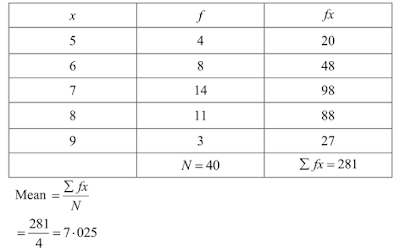2. Find the mean of the following data:
x: 19 21 23 25 27 29 31
f.  13 15 16 18 16 15 1

Solution3. If the mean of the following data is 20.6. Find the value of p.
x: 10 15 p 25 35
f:  3 10 25 7 5

Solution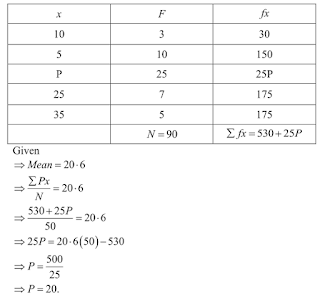4. If the mean of the following data is 15, find p.
x: 5 10 15 20 25
f: 6 p 6 10 5

Solution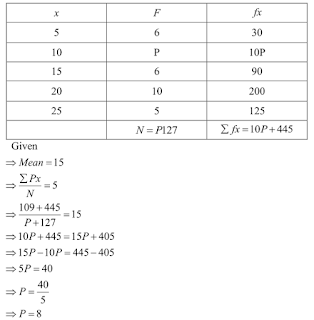5. Find the value of p for the following distribution whose mean is 16.6
x: 8  12  15  p  20  25 30
f. 12 16 20 24 16 8 4

Solution6. Find the missing value of p for the following distribution whose mean is 12.58 .
x: 5 8 10 12  p 20 25
f. 2 5 8 22 7 4 2

Solution7. Find the missing frequency (p) for the following distribution whose mean is 7.68.
x: 3 5 7 9 11 13
f: 6 8 15 p 8 4

Solution8. The following table gives the number of boys of a particular age in a class of 40 students.
Calculate the mean age of the students
Age (in years): 15 16 17 18 19 20
No. of students: 3  8   10 10  5  4

Solution9. Candidates of four schools appear in a mathematics test. The data were as follows:If the average score of the candidates of all the four schools is 66, find the number of
candidates that appeared from school III.

Solution10. Five coins were simultaneously tossed 1000 times and at each toss the number of heads
were observed. The number of tosses during which 0, 1, 2, 3, 4 and 5 heads were obtained
are shown in the table below. Find the mean number of heads per toss.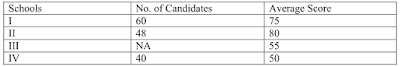Solution11. The arithmetic mean of the following data is 14. Find the value of k
xi : 5 10 15 20 25
fi : 7 k 8 4 5

Solution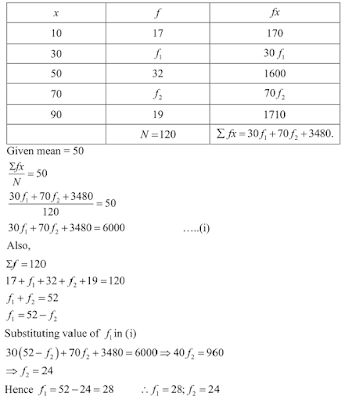12. The arithmetic mean of the following data is 25, find the value of k.
xi : 5 15 25 35 45
fi : 3 k 3 6 2

Solution13.  If the mean of the following data is 18.75. Find the value of p.
xi : 10 15 p 25 30
fi : 5 10 7 8 2

Solution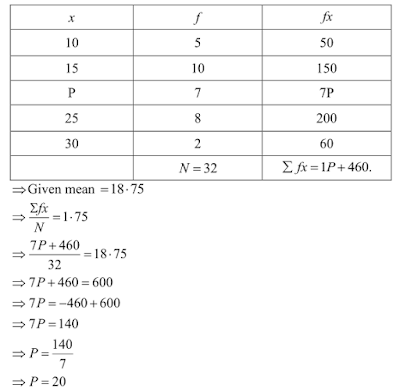14. Find the value of p, if the mean of the following distribution is 20.
x: 15 17 19 20+p 23
f: 2 3 4 5p 6

Solution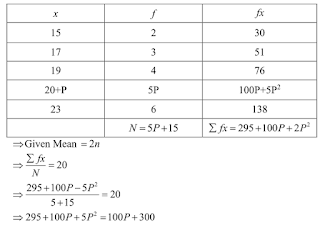15. Find the missing frequencies in the following frequency distribution if it is known that the
mean of the distribution is 50.
x: 10 30 50 70 90
f:  17 f1 32 f2 19

Solution